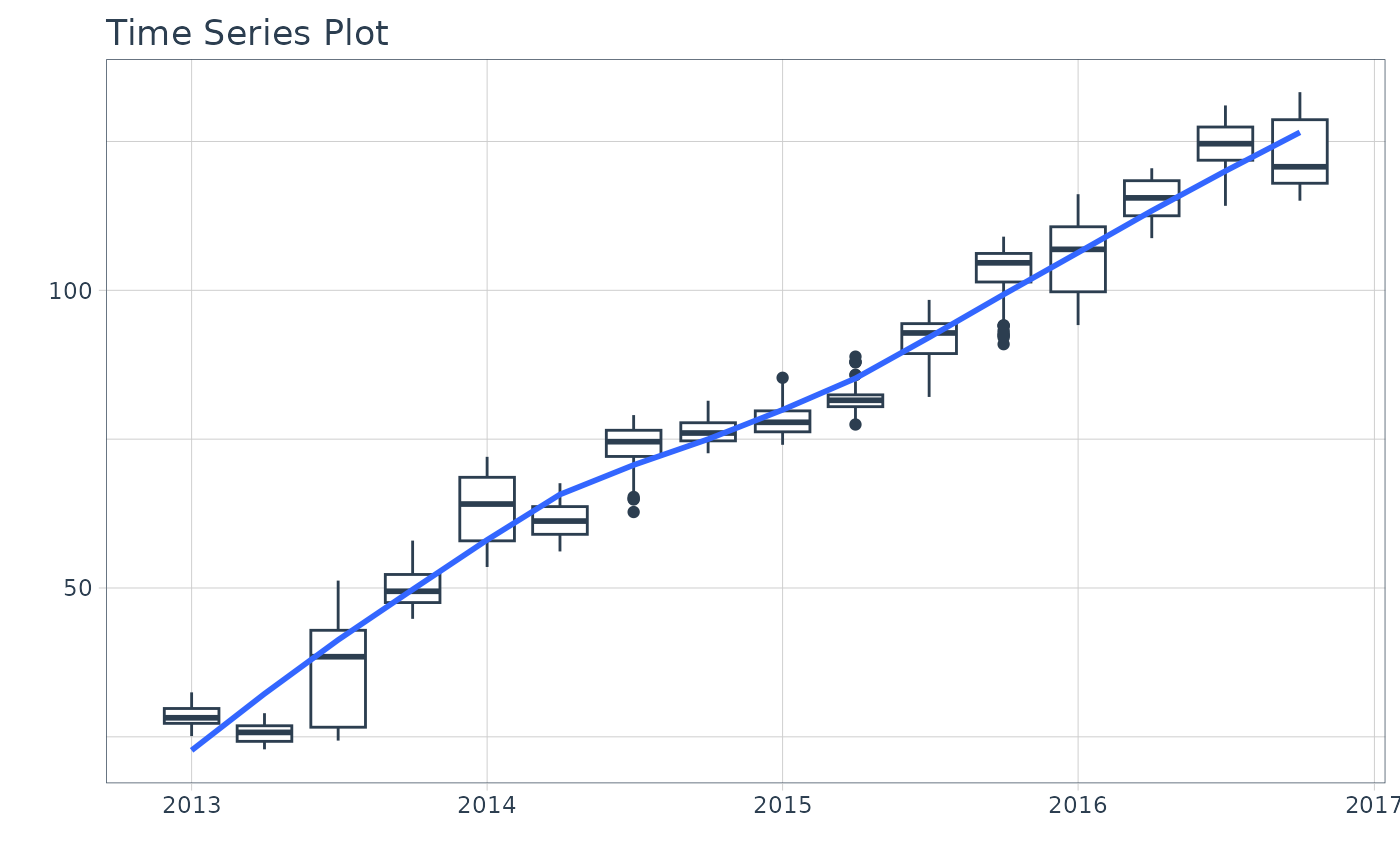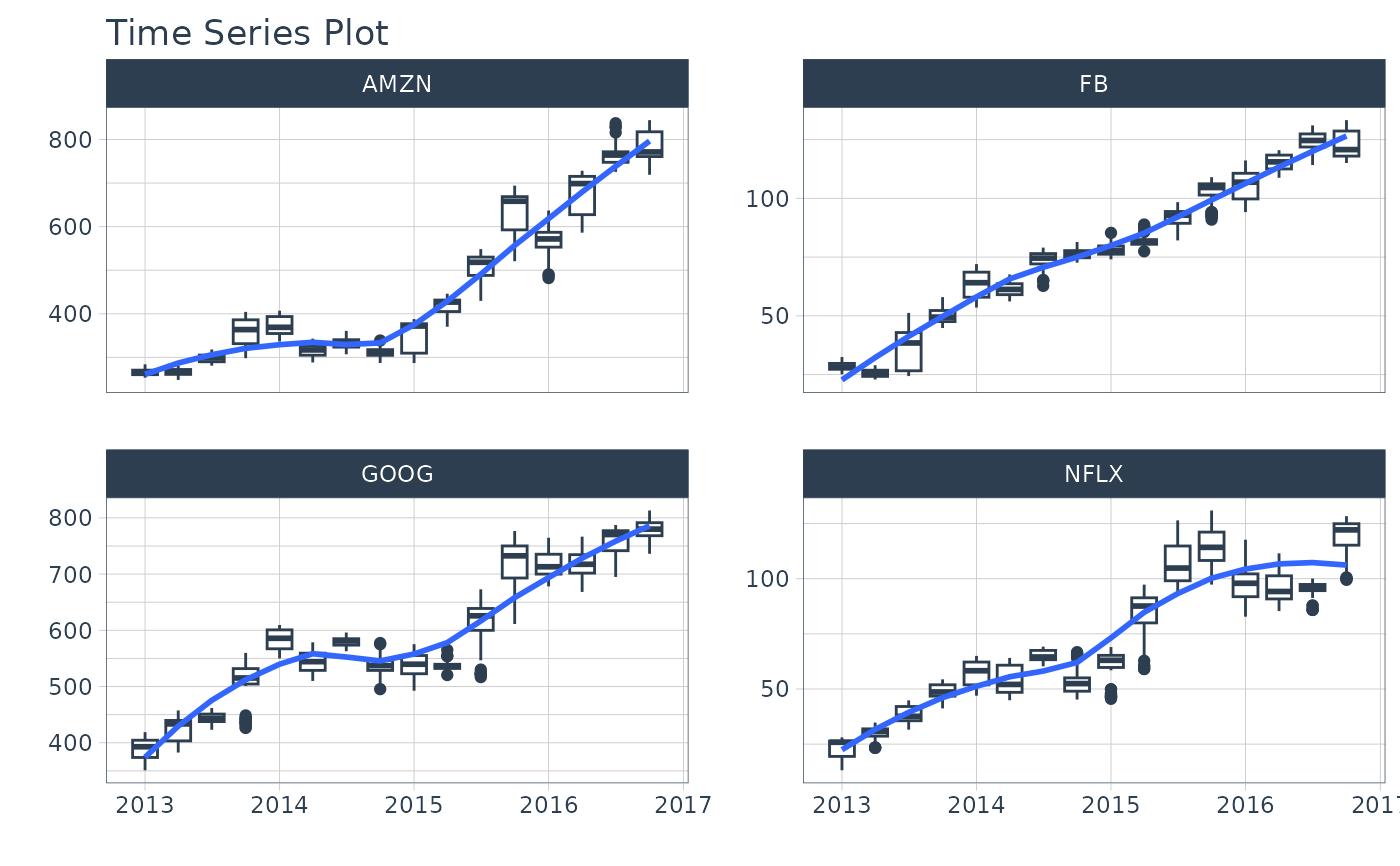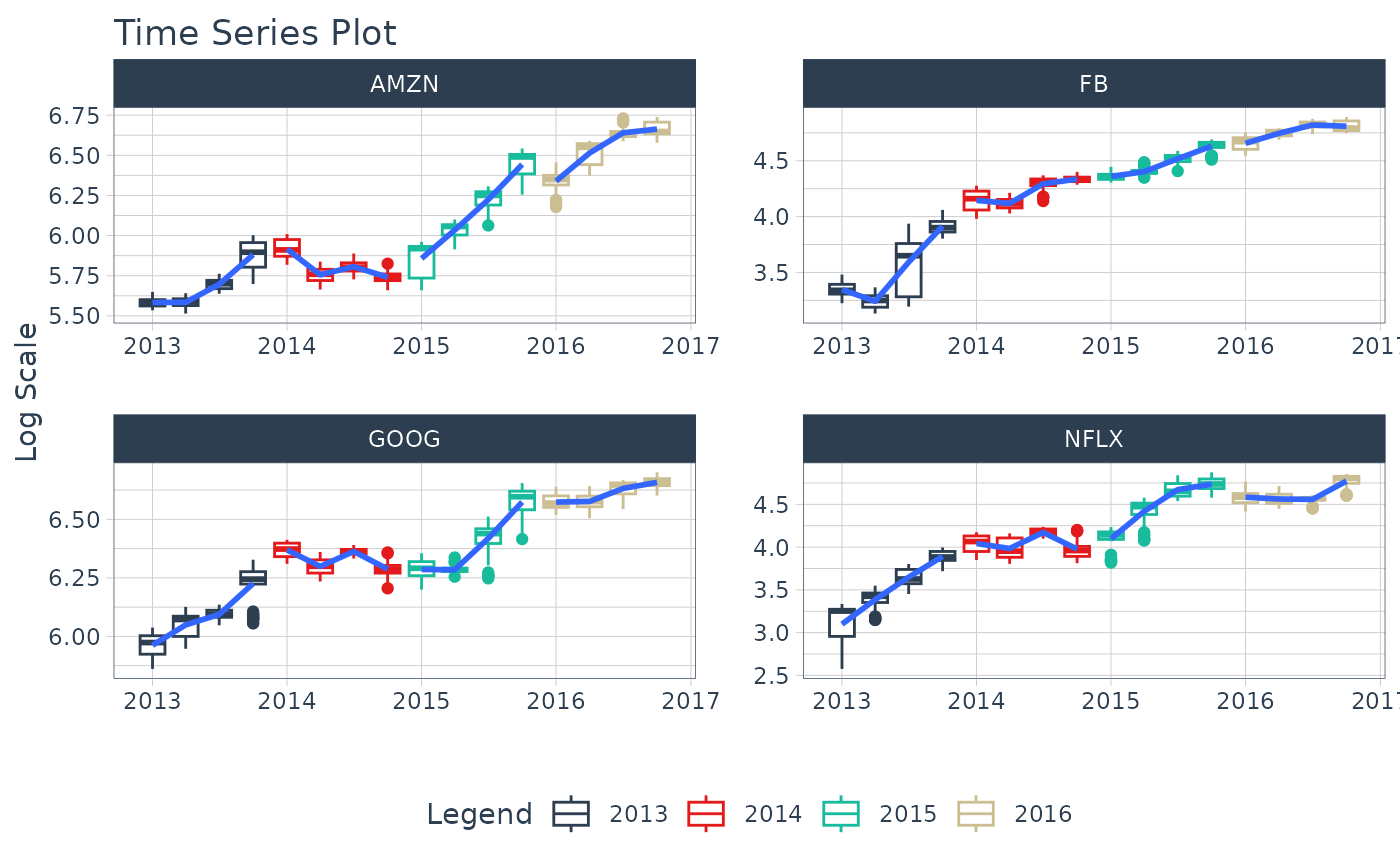A boxplot function that generates interactive plotly plots for time series.

## Usage

plot_time_series_boxplot(
.data,
.date_var,
.value,
.period,
.color_var = NULL,
.facet_vars = NULL,
.facet_ncol = 1,
.facet_nrow = 1,
.facet_scales = "free_y",
.facet_dir = "h",
.facet_collapse = FALSE,
.facet_collapse_sep = " ",
.facet_strip_remove = FALSE,
.line_color = "#2c3e50",
.line_size = 0.5,
.line_type = 1,
.line_alpha = 1,
.y_intercept = NULL,
.y_intercept_color = "#2c3e50",
.smooth = TRUE,
.smooth_func = ~mean(.x, na.rm = TRUE),
.smooth_period = "auto",
.smooth_message = FALSE,
.smooth_span = NULL,
.smooth_degree = 2,
.smooth_color = "#3366FF",
.smooth_size = 1,
.smooth_alpha = 1,
.legend_show = TRUE,
.title = "Time Series Plot",
.x_lab = "",
.y_lab = "",
.color_lab = "Legend",
.interactive = TRUE,
.plotly_slider = FALSE,
.trelliscope = FALSE,
.trelliscope_params = list()
)

## Arguments

.data

A tibble or data.frame with a time-based column

.date_var

A column containing either date or date-time values

.value

A column containing numeric values

.period

A time series unit of aggregation for the boxplot. Examples include:

• "1 week"

• "3 years"

• "30 minutes"

.color_var

A categorical column that can be used to change the line color

.facet_vars

One or more grouping columns that broken out into ggplot2 facets. These can be selected using tidyselect() helpers (e.g contains()).

.facet_ncol

Number of facet columns.

.facet_nrow

Number of facet rows (only used for .trelliscope = TRUE)

.facet_scales

Control facet x & y-axis ranges. Options include "fixed", "free", "free_y", "free_x"

.facet_dir

The direction of faceting ("h" for horizontal, "v" for vertical). Default is "h".

.facet_collapse

Multiple facets included on one facet strip instead of multiple facet strips.

.facet_collapse_sep

The separator used for collapsing facets.

.facet_strip_remove

Whether or not to remove the strip and text label for each facet.

.line_color

Line color. Overrided if .color_var is specified.

.line_size

Line size.

.line_type

Line type.

.line_alpha

Line alpha (opacity). Range: (0, 1).

.y_intercept

Value for a y-intercept on the plot

.y_intercept_color

Color for the y-intercept

.smooth

Logical - Whether or not to include a trendline smoother. Uses See smooth_vec() to apply a LOESS smoother.

.smooth_func

Defines how to aggregate the .value to show the smoothed trendline. The default is ~ mean(.x, na.rm = TRUE), which uses lambda function to ensure NA values are removed. Possible values are:

• A function, e.g. mean.

• A purrr-style lambda, e.g. ~ mean(.x, na.rm = TRUE)

.smooth_period

Number of observations to include in the Loess Smoother. Set to "auto" by default, which uses tk_get_trend() to determine a logical trend cycle.

.smooth_message

Logical. Whether or not to return the trend selected as a message. Useful for those that want to see what .smooth_period was selected.

.smooth_span

Percentage of observations to include in the Loess Smoother. You can use either period or span. See smooth_vec().

.smooth_degree

Flexibility of Loess Polynomial. Either 0, 1, 2 (0 = lest flexible, 2 = more flexible).

.smooth_color

Smoother line color

.smooth_size

Smoother line size

.smooth_alpha

Smoother alpha (opacity). Range: (0, 1).

.legend_show

Toggles on/off the Legend

.title

Title for the plot

.x_lab

X-axis label for the plot

.y_lab

Y-axis label for the plot

.color_lab

Legend label if a color_var is used.

.interactive

Returns either a static (ggplot2) visualization or an interactive (plotly) visualization

.plotly_slider

If TRUE, returns a plotly date range slider.

.trelliscope

Returns either a normal plot or a trelliscopejs plot (great for many time series) Must have trelliscopejs installed.

.trelliscope_params

Pass parameters to the trelliscopejs::facet_trelliscope() function as a list(). The only parameters that cannot be passed are:

• ncol: use .facet_ncol

• nrow: use .facet_nrow

• scales: use facet_scales

• as_plotly: use .interactive

## Value

A static ggplot2 plot or an interactive plotly plot

## Details

plot_time_series_boxplot() is a scalable function that works with both ungrouped and grouped data.frame objects (and tibbles!).

Interactive by Default

plot_time_series_boxplot() is built for exploration using:

• Interactive Plots: plotly (default) - Great for exploring!

• Static Plots: ggplot2 (set .interactive = FALSE) - Great for PDF Reports

By default, an interactive plotly visualization is returned.

Scalable with Facets & Dplyr Groups

plot_time_series_boxplot() returns multiple time series plots using ggplot2 facets:

• group_by() - If groups are detected, multiple facets are returned

• plot_time_series_boxplot(.facet_vars) - You can manually supply facets as well.

Can Transform Values just like ggplot

The .values argument accepts transformations just like ggplot2. For example, if you want to take the log of sales you can use a call like plot_time_series_boxplot(date, log(sales)) and the log transformation will be applied.

Smoother Period / Span Calculation

The .smooth = TRUE option returns a smoother that is calculated based on either:

1. A .smooth_func: The method of aggregation. Usually an aggregation like mean is used. The purrr-style function syntax can be used to apply complex functions.

2. A .smooth_period: Number of observations

3. A .smooth_span: A percentage of observations

By default, the .smooth_period is automatically calculated using 75% of the observertions. This is the same as geom_smooth(method = "loess", span = 0.75).

A user can specify a time-based window (e.g. .smooth_period = "1 year") or a numeric value (e.g. smooth_period = 365).

Time-based windows return the median number of observations in a window using tk_get_trend().

## Examples


library(dplyr)
library(tidyquant)
library(lubridate)
library(timetk)

# Works with individual time series
FANG %>%
filter(symbol == "FB") %>%
plot_time_series_boxplot(
.period      = "3 month",
.interactive = FALSE)# Works with groups
FANG %>%
group_by(symbol) %>%
plot_time_series_boxplot(
.period      = "3 months",
.facet_ncol  = 2,     # 2-column layout
.interactive = FALSE)if (FALSE) {
# Can also group inside & use .color_var
FANG %>%
mutate(year = year(date)) %>%
plot_time_series_boxplot(
.period      = "3 months",
.facet_vars   = c(symbol, year), # add groups/facets
.color_var    = year,            # color by year
.facet_ncol   = 4,
.facet_scales = "free",
.interactive  = FALSE)
}

# Can apply transformations to .value or .color_var
# - .color_var = year(date)
FANG %>%
plot_time_series_boxplot(
.period      = "3 months",
.color_var    = year(date),
.facet_vars   = contains("symbol"),
.facet_ncol   = 2,
.facet_scales = "free",
.y_lab        = "Log Scale",
.interactive  = FALSE)